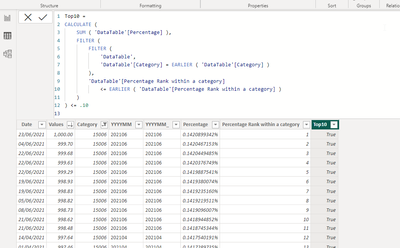cancel
Showing results for
Did you mean:Frequent Visitor

## Column flag for top percentage

Hello,

I need to create a calculated column where it crates a flag (yes/no) for the top 10% per category. Any saggestions?

To be more precise, i need to flag in a customer per store dataset, the top 10% customers per store, based on their spending.

2 REPLIES 2Super User

Hi @nikos_derv ,

This can by creating several calculated  columns but a warning, this looping in each row per category and can be very slow on  large table. I'd like to see a more efficient to this approach if there is.

``````Percentage =
//value percentage within a category
VAR CategoryTotal =
CALCULATE (
SUM ( 'DataTable'[Values] ),
ALLEXCEPT ( 'DataTable', 'DataTable'[Category] )
)
RETURN
DIVIDE ( 'DataTable'[Values], CategoryTotal )
``````
``````Percentage Rank within a category =
//rank the percentage with highest as one
RANKX (
FILTER (
'DataTable',
'DataTable'[Category] = EARLIER ( 'DataTable'[Category] )
),
'DataTable'[Values],
,
DESC,
DENSE
)
``````

The formula below can be very slow - might cause memory crash/webview2 error/bluescreen error.

``````Top10 =
//calculates the runninng percentage based on the rank and by category
//returns true if the running percentage is <= 10%
CALCULATE (
SUM ( 'DataTable'[Percentage] ),
FILTER (
FILTER (
'DataTable',
'DataTable'[Category] = EARLIER ( 'DataTable'[Category] )
),
'DataTable'[Percentage Rank within a category]
<= EARLIER ( 'DataTable'[Percentage Rank within a category] )
)
) <= .10
``````Please refer to the attached pbix. Go to the dataview then select Datatable table

Proud to be a Super User!

"Tell me and I’ll forget; show me and I may remember; involve me and I’ll understand."
Connect to me on LinkedIn || Need consulting? Hire me for a Power BI gig on UpWork.Frequent Visitor

I didn't get to use exactly your suggestion but you definately showed me the right direction.

In case anyone else might have the same issue, my solution was:

-create a column to calculate rank per category, using RankX (as mentioned above)

-create a column to calculate the max value of rank column, for each category

-final flag column: IF('rank column' <0.1 'max rank per category column' ,1,0)

Again, many thanks for our support!Announcements#### Exclusive opportunity for Women!

Join us for a free, hands-on Microsoft workshop led by women trainers for women where you will learn how to build a Dashboard in a Day!#### Power Platform Conference-Power BI and Fabric Sessions

Join us Oct 1 - 6 in Las Vegas for the Microsoft Power Platform Conference.Top Solution Authors
Top Kudoed Authors
Users online (1,840)